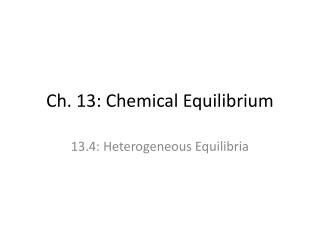Download PresentationCh. 13: Chemical Equilibrium

# Ch. 13: Chemical Equilibrium - PowerPoint PPT PresentationDownload Presentation## Ch. 13: Chemical Equilibrium

- - - - - - - - - - - - - - - - - - - - - - - - - - - E N D - - - - - - - - - - - - - - - - - - - - - - - - - - -
##### Presentation Transcript

1. Ch. 13: Chemical Equilibrium 13.4: Heterogeneous Equilibria

2. Heterogeneous Equilibria • involve more than one phase • position of heterogeneous equilibria does NOT depend on amounts of: • pure solids • pure liquids • because their concentrations stay constant (since they are PURE)

3. Heterogeneous Equilibria • do not include liquids or solids in equilibrium expression • only include gases and solutions (aq)

4. Example 1 • 2H2O(l) ⇄ 2H2(g) + O2(g) • 2H2O(g) ⇄ 2H2(g) + O2(g)

5. Ch. 13: Chemical Equilibrium 13.5/6: Applications of Equilibrium Constant (K)

6. Equilibrium Constant • if we know the value of K, we can predict: • tendency of a reaction to occur • if a set of concentrations could be at equilibrium • equilibrium position, given initial concentrations

7. Equilibrium Constant • If you start a reaction with only reactants: • concentration of reactants will decrease by a certain amount • concentration of products will increase by a same amount

8. Example 2 • The following reaction has a K of 16. You are starting reaction with 9 O3 molecules and 12 CO molecules. • Find the amount of each species at equilibrium. O3(g) + CO(g)  CO2(g) + O2(g)

9. Example 2

10. Example 2

11. Example 2

12. Example 2

13. Extent of a Reaction • If K >>1 • mostly products • goes essentially to completion • lies far to right • If K<< 1: • mostly reactants • reaction is negligible • lies far to left • size of K and time needed to reach equilibrium are NOT related • time required is determined by reaction rate (Ea)

14. Reaction Quotient • Q: equal to equilibrium expression but does not have to be at equilibrium • used to tell if a reaction is at equilibrium or not • relationship between Q and K tells which way the reaction will shift • Q=K: at equilibrium, no shift • Q > K: too large, forms reactants, shift to left • Q < K: too small, forms products, shift to right

15. Example 3 • For the synthesis of ammonia at 500°C, the equilibrium constant is 6.0 x 10-2. Predict the direction the system will shift to reach equilibrium in the following case: N2(g) + 3H2(g)  2NH3(g)

16. Example 3 • [NH3]0 = 1.0x10-3 M, [N2]0=1.0x10-5 M [H2]0=2.0x10-3 M Q > K so forms reactants, shifts to left

17. Example 4 • In the gas phase, dinitrogen tetroxide decomposes to gaseous nitrogen dioxide: N2O4(g) ⇄ 2NO2(g) • Consider an experiment in which gaseous N2O4 was placed in a flask and allowed to reach equilibrium at a T where KP = 0.133. At equilibrium, the pressure of N2O4 was found to be 2.71 atm. • Calculate the equilibrium pressure of NO2.

18. Example 4

19. Example 5 • At a certain temperature a 1.00 L flask initially contained 0.298 mol PCl3(g) and 8.70x10-3 mol PCl5(g). After the system had reached equilibrium, 2.00x10-3 mol Cl2(g) was found in the flask. PCl5(g)  PCl3(g) + Cl2(g) • Calculate the equilibrium concentrations of all the species and the value of K.

20. Example 5

21. Approximations • If K is very small, we can assume that the change (x) is going to be negligible • can be used to cancel out when adding or subtracting from a “normal” sized number • to simplify algebra 0

22. Example 6 • At 35°C, K=1.6x10-5 for the reaction 2NOCl(g) ⇄ 2NO(g) + Cl2(g) • Calculate the concentration of all species at equilibrium for the following mixtures • 2.0 mol NOCl in 2.0 L flask • 1.0 mol NOCl and 1.0 mol NO in 1.0 L flask • 2.0 mol NOCl and 1.0 mol Cl2 in 1.0 L flask

23. Example 6 • 2.0 mol NOCl in 2.0 L flask • [NOCl]=1.0 - (2 x 0.016)=0.97 M = 1.0 M, [NO]=0.032 M, [Cl2]=0.016 M

24. Example 6 • 1.0 mol NOCl and 1.0 mol NO in 1.0 L flask • [NOCl]=1.0 - (2x1.6x10-5)=0.999968 M = 1.0 M, [NO]=1.0 +(2x1.6x10-5)= 1.0 M, [Cl2]=1.6x10-5M

25. Example 6 • 2.0 mol NOCl and 1.0 mol Cl2 in 1.0 L flask • [NOCl]=2.0 - (2x4.0x10-3)=1.992 M = 2.0 M, [Cl2]=1.0+4.0x10-3=1.0 M, [NO]=0.0080 M,